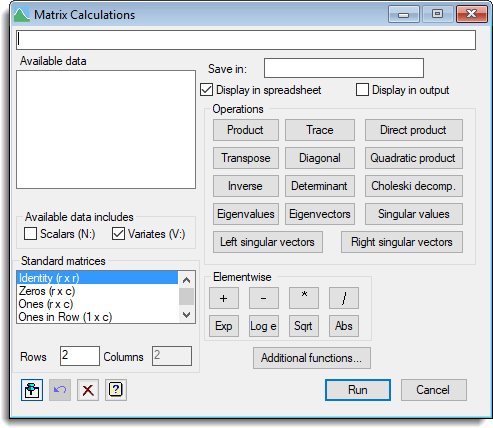1. Home
2. Matrix Calculations

# Matrix Calculations

Select menu: Data | Matrix Calculations

Use this to create an expression to perform a matrix calculation. The expression is displayed at the top of the menu and can be constructed step by step by combining data structures and operators. The operators displayed are specifically for matrices, but you can access more general functions using the Additional functions button. You can use brackets to control the order of evaluation when required. You can also type directly into the expression field. For more general calculations you can use the calculate menu.

1. After you have imported your data, from the menu select Data | Matrix Calculations.## Calculation

Provides a space to enter an expression for the calculation. This can be typed in directly or built up by clicking names in the Available data or Standard matrices lists together with operators or functions.

## Available data

This lists the matrices (rectangular, symmetric and diagonal) and their sizes (rows x columns) that are available for calculations. Rectangular, symmetric and diagonal matrices names are prefixed with M:, S: and D: respectively to display the type of each matrix. This list can be supplemented with variates and scalars using the Available data includes option. Double-clicking an identifier name will copy the name into the calculation field at the current cursor position.

## Save in

Specifies the name of the matrix (or scalar) to save the result from the calculation.

When selected, the matrix resulting from the calculation will be displayed in a spreadsheet.

## Display in output

When selected, the matrix resulting from the calculation will be printed in the Output window.

## Available data includes

These options allow scalars and variates to be displayed in the Available data list.

 Scalars (N:) Scalars will be displayed in the Available data list with the name prefixed with an N:. Variates (V:) Variates will be displayed in the Available data list with the name prefixed with a V:.

## Operations

These buttons specify various matrix operations. Clicking a button will place that function into the calculation list. If text is currently selected in the calculation field, then this will be inserted as the first argument of the function.

 Operation Genstat expression Definition Description Product A *+ B AB Matrix product or multiplication Trace TRACE(A) tr A The trace of a matrix. Direct product DPRODUCT(A;B) A Ä B Matrix direct product. Transpose T(A) A‘ Matrix transpose Diagonal DIAGONAL(A) Extract the diagonal of a matrix. Quadratic product T(A)*+X*+A A’XA Matrix quadratic product. Inverse INVERSE(A) A-1 The inverse of a matrix. Determinant DET(A) |A| The determinant of a matrix. Choleski Decomp. CHOLESKI(A) L: A = LL‘ The Choleski decomposition of a matrix. Eigenvalues EVALUES(A) The eigenvalues of a matrix. Eigenvectors EVECTORS(A) The eigenvectors of a matrix. Singular values SVALUES(A) The singular values of a matrix. Left singular vectors LSVECTORS(A) The left singular vectors of a matrix. Right singular vectors RSVECTORS(A) The right singular vectors of a matrix.

## Standard matrices

This lists various standard matrices that are available for calculations. The size of the standard matrix is specified in the Rows and Columns fields. Double-clicking a matrix type in the list will copy the name to the calculation field at the current cursor position.

## Elementwise

These buttons specify various scalar operations that will be applied to each element of the matrix. Clicking the button will place the function into the calculation list. If text is currently selected in the calculation field, then this will be inserted as the first argument of the function.

 Operation Description + Elementwise addition. – Elementwise subtraction. * Elementwise multiplication. / Elementwise division. Exp Elementwise exponential function. Log e Elementwise natural logarithm. Sqrt Elementwise square root. Abs Elementwise absolute value.

Note that the dimensions of the two matrices must match exactly for the operators +, -, * and /.Pin Controls whether to keep the dialog open when you click Run. When the pin is downthe dialog will remain open, otherwise when the pin is upthe dialog will close.Restore Restore names into edit fields and default settings.Clear Clear all fields and list boxes.Help Open the Help topic for this dialog.